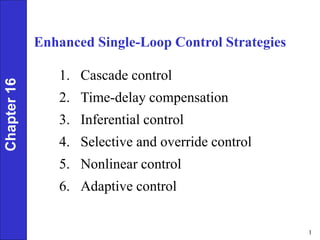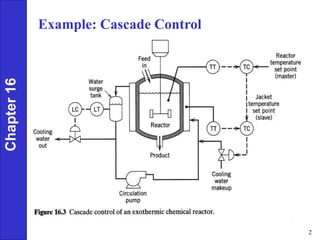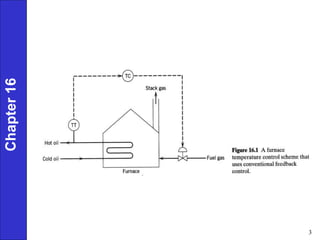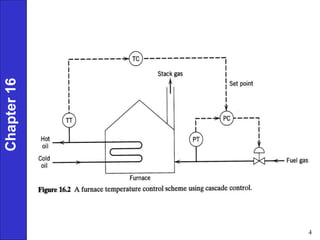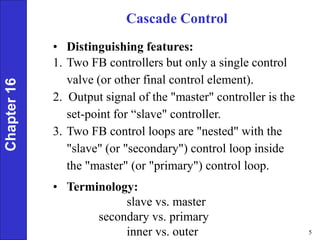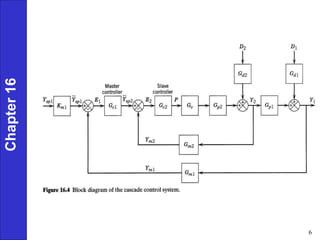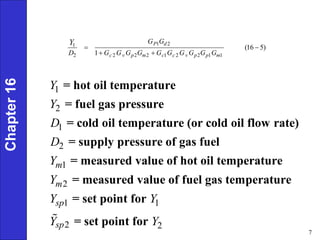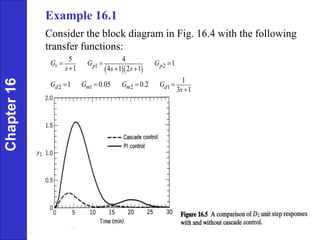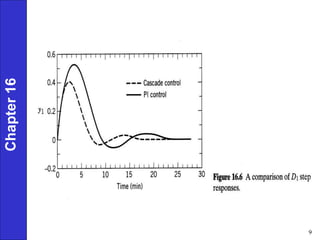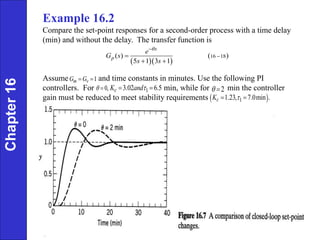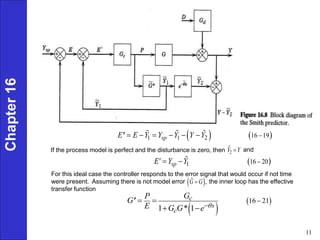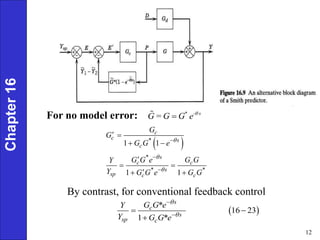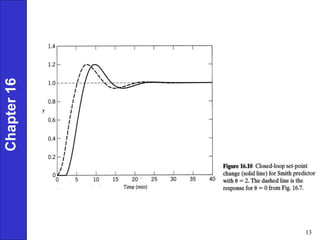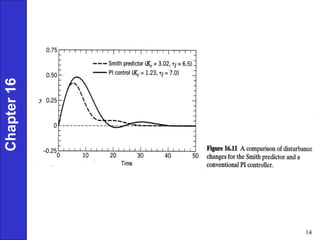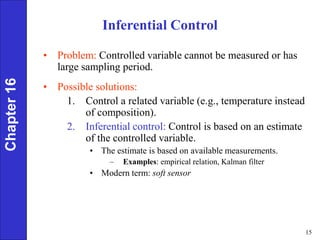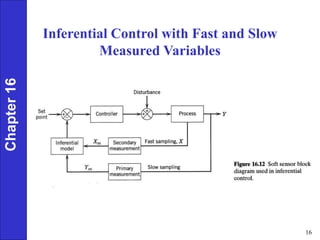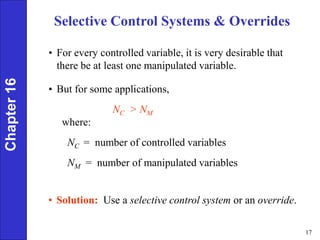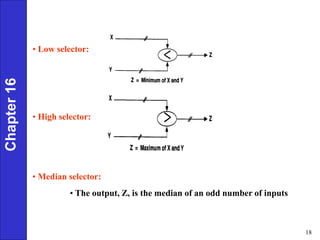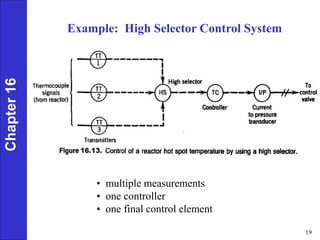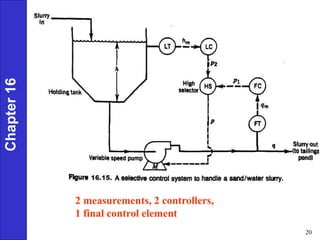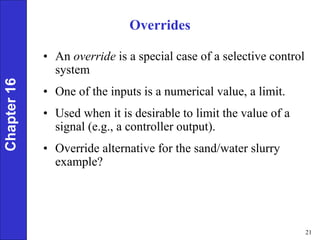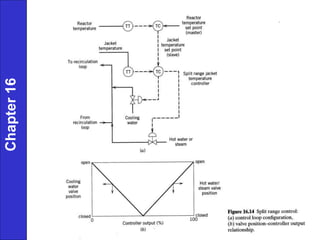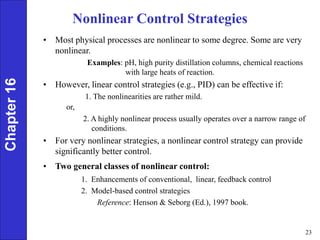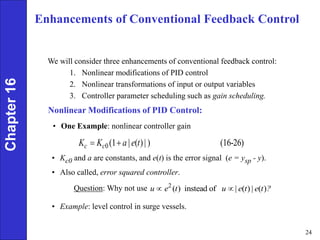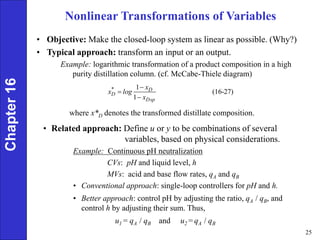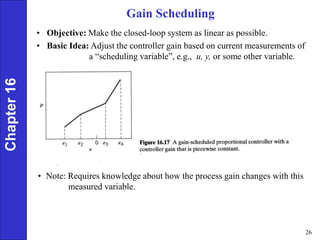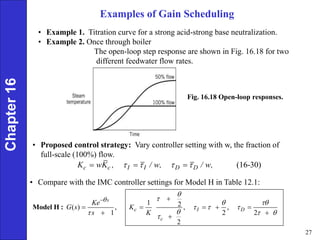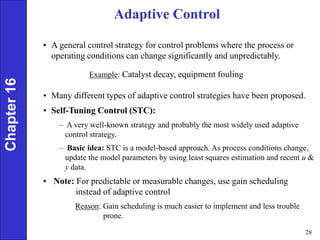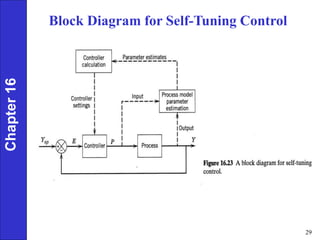1 de 29

### Ch16_1_27_05.ppt

1. 1 Enhanced Single-Loop Control Strategies 1. Cascade control 2. Time-delay compensation 3. Inferential control 4. Selective and override control 5. Nonlinear control 6. Adaptive control Chapter 16
2. 2 Example: Cascade Control Chapter 16
3. 3 Chapter 16
4. 4 Chapter 16
5. 5 Cascade Control • Distinguishing features: 1. Two FB controllers but only a single control valve (or other final control element). 2. Output signal of the "master" controller is the set-point for “slave" controller. 3. Two FB control loops are "nested" with the "slave" (or "secondary") control loop inside the "master" (or "primary") control loop. • Terminology: slave vs. master secondary vs. primary inner vs. outer Chapter 16
6. 6 Chapter 16
7. 7 1 2 1 2 1 2 = hot oil temperature = fuel gas pressure = cold oil temperature (or cold oil flow rate) = supply pressure of gas fuel = measured value of hot oil temperature = measured value of fuel gas tem m m Y Y D D Y Y 1 1 2 2 perature = set point for = set point for sp sp Y Y Y Y Chapter 16 1 2 1 2 2 2 2 1 2 2 1 1 (16 5) 1 P d c v p m c c v p p m G G D G G G G G G G G G G Y    
8. 8 Chapter 16 Example 16.1 Consider the block diagram in Fig. 16.4 with the following transfer functions:    1 2 1 2 2 1 5 4 1 1 4 1 2 1 1 1 0.05 0.2 3 1 v p p m m d d G G G s s s G G G G s           
9. 9 Chapter 16
10. 10 Chapter 16 Example 16.2 Compare the set-point responses for a second-order process with a time delay (min) and without the delay. The transfer function is Assume and time constants in minutes. Use the following PI controllers. For min, while for min the controller gain must be reduced to meet stability requirements      16 18 ( ) 5 1 3 1 s p e G s s s       1 m v G G   0,   1 3.02 6.5 c K and   2     1 1.23, 7.0min . c K   
11. 11 Chapter 16     1 1 2 16 19 sp E E Y Y Y Y Y        ' If the process model is perfect and the disturbance is zero, then 2 Y Y  and   1 16 20 sp E' Y Y    For this ideal case the controller responds to the error signal that would occur if not time were present. Assuming there is not model error the inner loop has the effective transfer function  , G G      16 21 1 * 1 c s c G P G E G G e        '
12. 12 For no model error: By contrast, for conventional feedback control   * - s G= G G e   1 1 1 1                 c c * s c * s c c * s * sp c c G G G G e G G e G G Y Y G G e G G Chapter 16   * 16 23 1 * s c s sp c G G e Y Y G G e       
13. 13 Chapter 16
14. 14 Chapter 16
15. 15 Chapter 16 Inferential Control • Problem: Controlled variable cannot be measured or has large sampling period. • Possible solutions: 1. Control a related variable (e.g., temperature instead of composition). 2. Inferential control: Control is based on an estimate of the controlled variable. • The estimate is based on available measurements. – Examples: empirical relation, Kalman filter • Modern term: soft sensor
16. 16 Inferential Control with Fast and Slow Measured Variables Chapter 16
17. 17 Selective Control Systems & Overrides • For every controlled variable, it is very desirable that there be at least one manipulated variable. • But for some applications, NC > NM where: NC = number of controlled variables NM = number of manipulated variables Chapter 16 • Solution: Use a selective control system or an override.
18. 18 • Low selector: • High selector: Chapter 16 • Median selector: • The output, Z, is the median of an odd number of inputs
19. 19 • multiple measurements • one controller • one final control element Chapter 16 Example: High Selector Control System
20. 20 2 measurements, 2 controllers, 1 final control element Chapter 16
21. 21 Overrides • An override is a special case of a selective control system • One of the inputs is a numerical value, a limit. • Used when it is desirable to limit the value of a signal (e.g., a controller output). • Override alternative for the sand/water slurry example? Chapter 16
22. 22 Chapter 16
23. 23 Nonlinear Control Strategies • Most physical processes are nonlinear to some degree. Some are very nonlinear. Examples: pH, high purity distillation columns, chemical reactions with large heats of reaction. • However, linear control strategies (e.g., PID) can be effective if: 1. The nonlinearities are rather mild. or, 2. A highly nonlinear process usually operates over a narrow range of conditions. • For very nonlinear strategies, a nonlinear control strategy can provide significantly better control. • Two general classes of nonlinear control: 1. Enhancements of conventional, linear, feedback control 2. Model-based control strategies Reference: Henson & Seborg (Ed.), 1997 book. Chapter 16
24. 24 Enhancements of Conventional Feedback Control We will consider three enhancements of conventional feedback control: 1. Nonlinear modifications of PID control 2. Nonlinear transformations of input or output variables 3. Controller parameter scheduling such as gain scheduling. Nonlinear Modifications of PID Control: 0(1 ( ) ) (16-26) c c K K a|e t |   Chapter 16 • Kc0 and a are constants, and e(t) is the error signal (e = ysp - y). • Also called, error squared controller. Question: Why not use • Example: level control in surge vessels. • One Example: nonlinear controller gain 2 ( ) instead of ( ) ( ) u e t u |e t |e t ?  
25. 25 Nonlinear Transformations of Variables • Objective: Make the closed-loop system as linear as possible. (Why?) • Typical approach: transform an input or an output. Example: logarithmic transformation of a product composition in a high purity distillation column. (cf. McCabe-Thiele diagram) Chapter 16 where x*D denotes the transformed distillate composition. • Related approach: Define u or y to be combinations of several variables, based on physical considerations. Example: Continuous pH neutralization CVs: pH and liquid level, h MVs: acid and base flow rates, qA and qB • Conventional approach: single-loop controllers for pH and h. • Better approach: control pH by adjusting the ratio, qA / qB, and control h by adjusting their sum. Thus, u1 = qA / qB and u2 = qA / qB 1 (16-27) 1 * D D Dsp x x log x   
26. 26 Gain Scheduling • Objective: Make the closed-loop system as linear as possible. • Basic Idea: Adjust the controller gain based on current measurements of a “scheduling variable”, e.g., u, y, or some other variable. Chapter 16 • Note: Requires knowledge about how the process gain changes with this measured variable.
27. 27 Chapter 16 Examples of Gain Scheduling • Example 1. Titration curve for a strong acid-strong base neutralization. • Example 2. Once through boiler The open-loop step response are shown in Fig. 16.18 for two different feedwater flow rates. Fig. 16.18 Open-loop responses. • Proposed control strategy: Vary controller setting with w, the fraction of full-scale (100%) flow. (16-30) c c I I D D K wK , / w, / w,        • Compare with the IMC controller settings for Model H in Table 12.1: 1 2 ( ) , , , 1 2 2 2 s c I D c Ke G s K s K                        Model H :
28. 28 Chapter 16 Adaptive Control • A general control strategy for control problems where the process or operating conditions can change significantly and unpredictably. Example: Catalyst decay, equipment fouling • Many different types of adaptive control strategies have been proposed. • Self-Tuning Control (STC): – A very well-known strategy and probably the most widely used adaptive control strategy. – Basic idea: STC is a model-based approach. As process conditions change, update the model parameters by using least squares estimation and recent u & y data. • Note: For predictable or measurable changes, use gain scheduling instead of adaptive control Reason: Gain scheduling is much easier to implement and less trouble prone.
29. 29 Chapter 16 Block Diagram for Self-Tuning Control